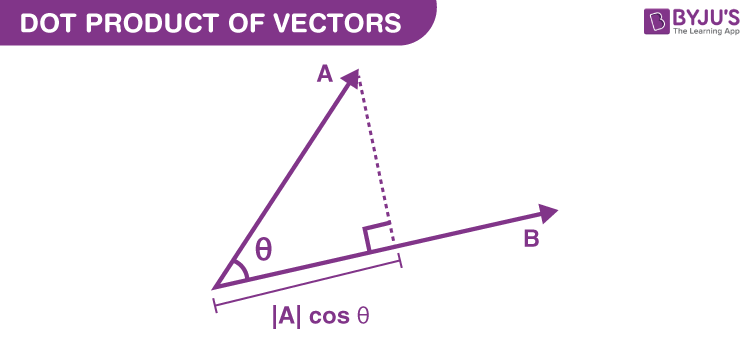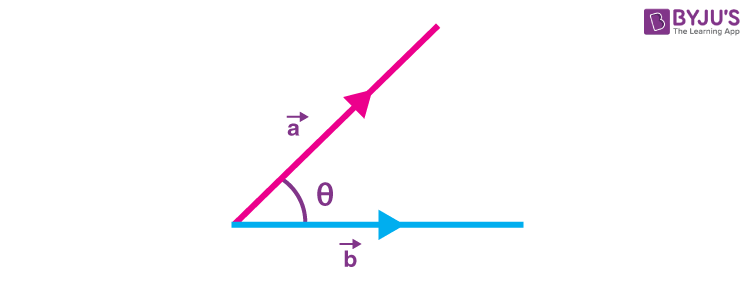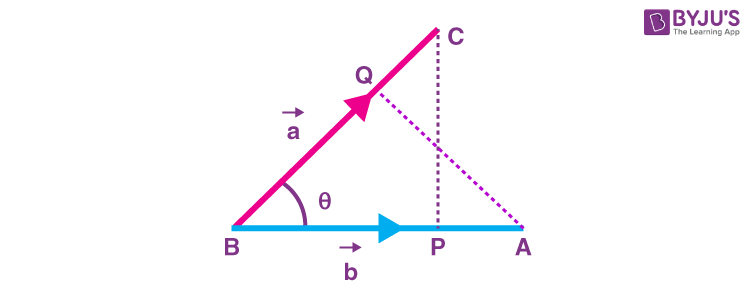# Dot Product Of Two Vectors

Vector is a quantity that has both magnitude and direction. Some mathematical operations can be performed on vectors such as addition and multiplication. The multiplication of vectors can be done in two ways, i.e. dot product and cross product. In this article, you will learn the dot product of two vectors with the help of examples.

The definition of dot product can be given in two ways, i.e. algebraically and geometrically. Algebraically, the dot product is defined as the sum of the products of the corresponding entries of the two sequences of numbers. Geometrically, it is the product of the two vectors’ Euclidean magnitudes and the cosine of the angle between them. Both the definitions are equivalent when working with Cartesian coordinates. However, the dot product of two vectors is the product of the magnitude of the two vectors and the cos of the angle between them. To recall, vectors are multiplied using two methods

• scalar product of vectors or dot product
• vector product of vectors or cross product

The difference between both the methods is just that, using the first method, we get a scalar value as resultant and using the second technique the value obtained is again a vector in nature.

## Dot Product of VectorsThe scalar product of two vectors a and b of magnitude |a| and |b| is given as |a||b| cos θ, where θ represents the angle between the vectors a and b taken in the direction of the vectors.
We can express the scalar product as:

a.b=|a||b| cosθ

where |a| and |b| represent the magnitude of the vectors a and while cos θ denotes the cosine of the angle between both the vectors and a.b indicate the dot product of the two vectors.In the case, where any of the vectors is zero, the angle θ is not defined and in such a scenario a.b is given as zero.

### Projection of VectorsBP is known to be the projection of a vector a on vector b in the direction of vector b given by |a| cos θ.

Similarly, the projection of vector b on a vector a in the direction of the vector a is given by |b| cos θ.

Projection of vector a in direction of vector b is expressed as

$$\begin{array}{l} BP = \frac{a.b}{|b|}\end{array}$$

$$\begin{array}{l}\Rightarrow \overrightarrow{BP} = \frac{a.b}{|b|} × \hat{b}\end{array}$$

$$\begin{array}{l}\Rightarrow \overrightarrow{BP} = \frac{a.b}{|b|}.\frac{b}{|b|}\end{array}$$
$$\begin{array}{l}\Rightarrow \overrightarrow{BP} =\frac{a.b}{|b|^2}b\end{array}$$

Similarly, projection of vector b in direction of vector a is expressed as

$$\begin{array}{l}BQ = \frac{a.b}{|a|}\end{array}$$
$$\begin{array}{l}\Rightarrow \overrightarrow{BQ} = \frac{a.b}{|a|} \times \hat{a}\end{array}$$
$$\begin{array}{l}\Rightarrow \overrightarrow{BQ} = \frac{a.b}{|a|} \frac{a}{|a|}\end{array}$$
$$\begin{array}{l}\Rightarrow \overrightarrow{BQ} = \frac{a.b}{|a|^2}a\end{array}$$

Thus, we see that the dot product of two vectors is the product of magnitude of one vector with the resolved component of the other in the direction of the first vector.

### Dot Product Properties of Vector:

• Property 1: Dot product of two vectors is commutative i.e. a.b = b.a = ab cos θ.
• Property 2: If a.b = 0 then it can be clearly seen that either b or a is zero or cos θ = 0.
$$\begin{array}{l}\Rightarrow \theta=\frac{\pi}{2}.\end{array}$$
It suggests that either of the vectors is zero or they are perpendicular to each other.
• Property 3: Also we know that using scalar product of vectors (pa).(qb)=(pb).(qa)=pq a.b
• Property 4: The dot product of a vector to itself is the magnitude squared of the vector i.e. a.a = a.a  cos 0 = a2
• Property 5: The dot product follows the distributive law also i.e. a.(b + c) = a.b + a.c
• Property 6: In terms of orthogonal coordinates for mutually perpendicular vectors it is seen that
$$\begin{array}{l}\hat{i}.\hat{i} = \hat{j}.\hat{j}= \hat{k}.\hat{k} = 1\end{array}$$
• Property 7: In terms of unit vectors, if
$$\begin{array}{l}a = a_{1}\hat{i}+ a_{2}\hat{j}+a_{3}\hat{k} \ and\ b = b_{1}\hat{i}+ b_{2}\hat{j}+b_{3}\hat{k}\end{array}$$
then
$$\begin{array}{l}a.b = (a_1 \hat{i} + a_2 \hat{j} + a_3 \hat{k}).(b_1 \hat{i} + b_2 \hat{j} + b_3 \hat{k})\end{array}$$
$$\begin{array}{l}\Rightarrow a_1b_1 + a_2b_2 + a_3b_3 = ab\ cos\theta\end{array}$$

Also Check: Vector product

### Dot Product of Two Vectors Example Questions

Example 1: Let there be two vectors [6, 2, -1] and [5, -8, 2]. Find the dot product of the vectors.

Solution:

Given vectors: [6, 2, -1] and [5, -8, 2] be a and b respectively.

a.b = (6)(5) + (2)(-8) + (-1)(2)

a.b = 30 – 16 – 2

a.b = 12

Example 2: Let there be two vectors |a|=4 and |b|=2 and θ = 60°. Find their dot product.

Solution:

a.b = |a||b|cos θ

a.b = 4.2 cos 60°

a.b = 4.2 × (1/2)

a.b = 4